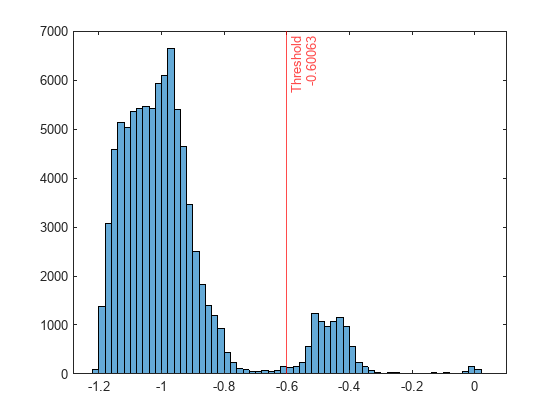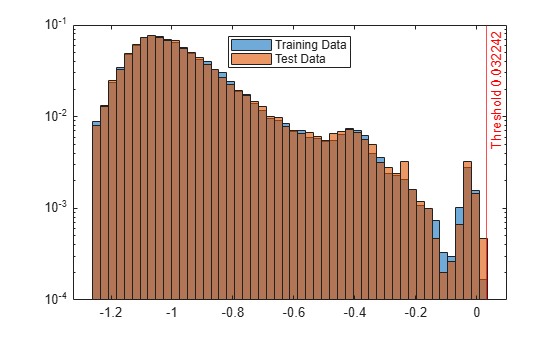# OneClassSVM

One-class support vector machine (SVM) for anomaly detection

Since R2022b

## Description

Use a one-class support vector machine model object `OneClassSVM` for outlier detection and novelty detection.

• Outlier detection (detecting anomalies in training data) — Detect anomalies in training data by using the `ocsvm` function. The `ocsvm` function trains a `OneClassSVM` object and returns anomaly indicators and scores for the training data.

• Novelty detection (detecting anomalies in new data with uncontaminated training data) — Create a `OneClassSVM` object by passing uncontaminated training data (data with no outliers) to `ocsvm`, and detect anomalies in new data by passing the object and the new data to the object function `isanomaly`. The `isanomaly` function returns anomaly indicators and scores for the new data.

## Creation

Create a `OneClassSVM` object by using the `ocsvm` function.

## Properties

expand all

Categorical predictor indices, specified as a vector of positive integers. `CategoricalPredictors` contains index values indicating that the corresponding predictors are categorical. The index values are between 1 and `p`, where `p` is the number of predictors used to train the model. If none of the predictors are categorical, then this property is empty (`[]`).

Fraction of anomalies in the training data, specified as a numeric scalar in the range `[0,1]`.

• If the `ContaminationFraction` value is 0, then `ocsvm` treats all training observations as normal observations, and sets the score threshold (`ScoreThreshold` property value) to the maximum anomaly score value of the training data.

• If the `ContaminationFraction` value is in the range (`0`,`1`], then `ocsvm` determines the threshold value (`ScoreThreshold` property value) so that the function detects the specified fraction of training observations as anomalies.

Kernel scale parameter, specified as a positive scalar.

Regularization term strength, specified as a nonnegative scalar.

Predictor means of the training data, specified as a numeric vector.

• If you specify `StandardizeData=true` when you train a one-class SVM model using `ocsvm`:

• The `ocsvm` function does not standardize columns that contain categorical variables. The elements in `Mu` for categorical variables contain `NaN` values.

• The `isanomaly` function standardizes the input data by using the predictor means in `Mu` and standard deviations in `Sigma`.

The length of `Mu` is equal to the number of predictors.

• If you set `StandardizeData=false`, then `Mu` is an empty vector (`[]`).

Number of dimensions of the expanded space, specified as a positive integer.

Value of the objective function that the Limited-memory Broyden-Fletcher-Goldfarb-Shanno (LBFGS) solver minimizes to solve the one-class SVM problem, specified as a scalar.

Predictor variable names, specified as a cell array of character vectors. The order of the elements in `PredictorNames` corresponds to the order in which the predictor names appear in the training data.

Threshold for the anomaly score used to identify anomalies in the training data, specified as a numeric scalar in the range `(–Inf,Inf)`.

The software identifies observations with anomaly scores above the threshold as anomalies.

Predictor standard deviations of the training data, specified as a numeric vector.

• If you specify `StandardizeData=true` when you train a one-class SVM model using `ocsvm`:

• The `ocsvm` function does not standardize columns that contain categorical variables. The elements in `Sigma` for categorical variables contain `NaN` values.

• The `isanomaly` function standardizes the input data by using the predictor means in `Mu` and standard deviations in `Sigma`.

The length of `Sigma` is equal to the number of predictors.

• If you set `StandardizeData=false`, then `Sigma` is an empty vector (`[]`).

## Object Functions

 `isanomaly` Find anomalies in data using one-class support vector machine (SVM)

## Examples

collapse all

Detect outliers (anomalies in training data) by using the `ocsvm` function.

Load the sample data set `NYCHousing2015`.

`load NYCHousing2015`

The data set includes 10 variables with information on the sales of properties in New York City in 2015. Display a summary of the data set.

`summary(NYCHousing2015)`
```double Values: Min 1 Median 3 Max 5 NEIGHBORHOOD: 91446×1 cell array of character vectors BUILDINGCLASSCATEGORY: 91446×1 cell array of character vectors RESIDENTIALUNITS: 91446×1 double Values: Min 0 Median 1 Max 8759 COMMERCIALUNITS: 91446×1 double Values: Min 0 Median 0 Max 612 LANDSQUAREFEET: 91446×1 double Values: Min 0 Median 1700 Max 2.9306e+07 GROSSSQUAREFEET: 91446×1 double Values: Min 0 Median 1056 Max 8.9422e+06 YEARBUILT: 91446×1 double Values: Min 0 Median 1939 Max 2016 SALEPRICE: 91446×1 double Values: Min 0 Median 3.3333e+05 Max 4.1111e+09 SALEDATE: 91446×1 datetime Values: Min 01-Jan-2015 Median 09-Jul-2015 Max 31-Dec-2015 ```

The `SALEDATE` column is a `datetime` array, which is not supported by `ocsvm`. Create columns for the month and day numbers of the `datetime` values, and delete the `SALEDATE` column.

```[~,NYCHousing2015.MM,NYCHousing2015.DD] = ymd(NYCHousing2015.SALEDATE); NYCHousing2015.SALEDATE = [];```

Train a one-class SVM model for `NYCHousing2015`. Specify the fraction of anomalies in the training observations as 0.1, and specify the first variable (`BOROUGH`) as a categorical predictor. The first variable is a numeric array, so `ocsvm` assumes it is a continuous variable unless you specify the variable as a categorical variable. In addition, specify `StandardizeData` as `true` to standardize the input data, because the predictors have largely different scales. Set `KernelScale` to `"auto"` so that the software selects an appropriate kernel scale parameter using a heuristic procedure.

```rng("default") % For reproducibility [Mdl,tf,scores] = ocsvm(NYCHousing2015,ContaminationFraction=0.1, ... CategoricalPredictors=1,StandardizeData=true, ... KernelScale="auto");```

`Mdl` is a `OneClassSVM` object. `ocsvm` also returns the anomaly indicators (`tf`) and anomaly scores (`scores`) for the training data `NYCHousing2015`.

Plot a histogram of the score values. Create a vertical line at the score threshold corresponding to the specified fraction.

```histogram(scores) xline(Mdl.ScoreThreshold,"r-",["Threshold" Mdl.ScoreThreshold])```If you want to identify anomalies with a different contamination fraction (for example, 0.01), you can train a new one-class SVM model.

```rng("default") % For reproducibility [newMdl,newtf,scores] = ocsvm(NYCHousing2015, ... ContaminationFraction=0.01,CategoricalPredictors=1, ... KernelScale="auto"); ```

If you want to identify anomalies with a different score threshold value (for example, -0.7), you can pass the `OneClassSVM` object, the training data, and a new threshold value to the `isanomaly` function.

```[newtf,scores] = isanomaly(Mdl,NYCHousing2015,ScoreThreshold=-0.7); ```

Note that changing the contamination fraction or score threshold changes the anomaly indicators only, and does not affect the anomaly scores. Therefore, if you do not want to compute the anomaly scores again by using `ocsvm` or `isanomaly`, you can obtain a new anomaly indicator with the existing score values.

Change the fraction of anomalies in the training data to 0.01.

`newContaminationFraction = 0.01;`

Find a new score threshold by using the `quantile` function.

`newScoreThreshold = quantile(scores,1-newContaminationFraction)`
```newScoreThreshold = -0.3745 ```

Obtain a new anomaly indicator.

`newtf = scores > newScoreThreshold;`

Create a `OneClassSVM` object for uncontaminated training observations by using the `ocsvm` function. Then detect novelties (anomalies in new data) by passing the object and the new data to the object function `isanomaly`.

Load the 1994 census data stored in `census1994.mat`. The data set consists of demographic data from the US Census Bureau to predict whether an individual makes over \$50,000 per year.

`load census1994`

`census1994` contains the training data set `adultdata` and the test data set `adulttest`.

`ocsvm` does not use observations with missing values. Remove missing values in the data sets to reduce memory consumption and speed up training.

```adultdata = rmmissing(adultdata); adulttest = rmmissing(adulttest);```

Train a one-class SVM for `adultdata`. Assume that `adultdata` does not contain outliers. Specify `StandardizeData` as `true` to standardize the input data, and set `KernelScale` to `"auto"` to let the function select an appropriate kernel scale parameter using a heuristic procedure.

```rng("default") % For reproducibility [Mdl,~,s] = ocsvm(adultdata,StandardizeData=true,KernelScale="auto");```

`Mdl` is a `OneClassSVM` object. If you do not specify the `ContaminationFraction` name-value argument as a value greater than 0, then `ocsvm` treats all training observations as normal observations. The function sets the score threshold to the maximum score value. Display the threshold value.

`Mdl.ScoreThreshold`
```ans = 0.0322 ```

Find anomalies in `adulttest` by using the trained one-class SVM model. Because you specified `StandardizeData=true` when you trained the model, the `isanomaly` function standardizes the input data by using the predictor means and standard deviations of the training data stored in the `Mu` and `Sigma` properties, respectively.

`[tf_test,s_test] = isanomaly(Mdl,adulttest);`

The `isanomaly` function returns the anomaly indicators `tf_test` and scores `s_test` for `adulttest`. By default, `isanomaly` identifies observations with scores above the threshold (`Mdl.ScoreThreshold`) as anomalies.

Create histograms for the anomaly scores `s` and `s_test`. Create a vertical line at the threshold of the anomaly scores.

```h1 = histogram(s,NumBins=50,Normalization="probability"); hold on h2 = histogram(s_test,h1.BinEdges,Normalization="probability"); xline(Mdl.ScoreThreshold,"r-",join(["Threshold" Mdl.ScoreThreshold])) h1.Parent.YScale = 'log'; h2.Parent.YScale = 'log'; legend("Training Data","Test Data",Location="north") hold off```Display the observation index of the anomalies in the test data.

`find(tf_test)`
```ans = 0x1 empty double column vector ```

The anomaly score distribution of the test data is similar to that of the training data, so `isanomaly` does not detect any anomalies in the test data with the default threshold value. You can specify a different threshold value by using the `ScoreThreshold` name-value argument. For an example, see Specify Anomaly Score Threshold.